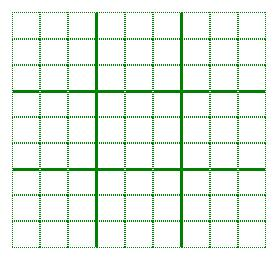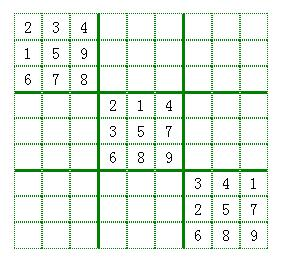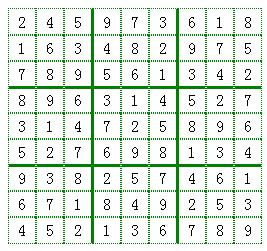11 21 31 41 51 61 71 81 91 12 22 32 42 52 62 72 82 92 13 23 33 43 53 63 73 83 93 14 24 34 44 54 64 74 84 94 15 25 35 45 55 65 75 85 95 16 26 36 46 56 66 76 86 96 17 27 37 47 57 67 77 87 97 18 28 38 48 58 68 78 88 98 19 29 39 49 59 69 79 89 99

…………

``````createSdArr:function(){
//生成数独数组。
var that = this;
try{
this.sdArr = [];
this.setThird(2,2);
this.setThird(5,5);
this.setThird(8,8);
var allNum = [1,2,3,4,5,6,7,8,9];
outerfor:
for(var i=1;i<=9;i++){
innerfor:
for(var j=1;j<=9;j++){
if(this.sdArr[parseInt(i+''+j)]){
continue innerfor;
}
var XArr = this.getXArr(j,this.sdArr);
var YArr = this.getYArr(i,this.sdArr);
var thArr = this.getThArr(i,j,this.sdArr);
var arr = getConnect(getConnect(XArr,YArr),thArr);
var ableArr = arrMinus(allNum,arr);

if(ableArr.length == 0){
this.createSdArr();
return;
break outerfor;
}

var item;
//如果生成的重复了就重新生成。
do{
item = ableArr[getRandom(ableArr.length)-1];
}while((\$.inArray(item, arr)>-1));

this.sdArr[parseInt(i+''+j)] = item;
}
}
this.backupSdArr = this.sdArr.slice();
}catch(e){
//如果因为超出浏览器的栈限制出错，就重新运行。
that.createSdArr();
}
},
getXArr:function(j,sdArr){
//获取所在行的值。
var arr = [];
for(var a =1;a<=9;a++){
if(this.sdArr[parseInt(a+""+j)]){
arr.push(sdArr[parseInt(a+""+j)])
}
}
return arr;
},
getYArr:function(i,sdArr){
//获取所在列的值。
var arr = [];
for(var a =1;a<=9;a++){
if(sdArr[parseInt(i+''+a)]){
arr.push(sdArr[parseInt(i+''+a)])
}
}
return arr;
},
getThArr:function(i,j,sdArr){
//获取所在三宫格的值。
var arr = [];
var cenNum = this.getTh(i,j);
var thIndexArr = [cenNum-11,cenNum-1,cenNum+9,cenNum-10,cenNum,cenNum+10,cenNum-9,cenNum+1,cenNum+11];
for(var a =0;a<9;a++){
if(sdArr[thIndexArr[a]]){
arr.push(sdArr[thIndexArr[a]]);
}
}
return arr;
},
getTh:function(i,j){
//获取所在三宫格的中间位坐标。
var cenArr = [22,52,82,25,55,85,28,58,88];
var index = (Math.ceil(j/3)-1) * 3 +Math.ceil(i/3) -1;
var cenNum = cenArr[index];
return cenNum;
},
setThird:function(i,j){
//为对角线上的三个三宫格随机生成。
var numArr = [1,2,3,4,5,6,7,8,9];
var sortedNumArr= numArr.sort(function(){return Math.random()-0.5>0?-1:1});
var cenNum = parseInt(i+''+j);
var thIndexArr = [cenNum-11,cenNum-1,cenNum+9,cenNum-10,cenNum,cenNum+10,cenNum-9,cenNum+1,cenNum+11];
for(var a=0;a<9;a++){
this.sdArr[thIndexArr[a]] = sortedNumArr[a];
}
}``````http://runningls.com/demos/2016/soduku/

https://github.com/liusaint/games/tree/master/soduku